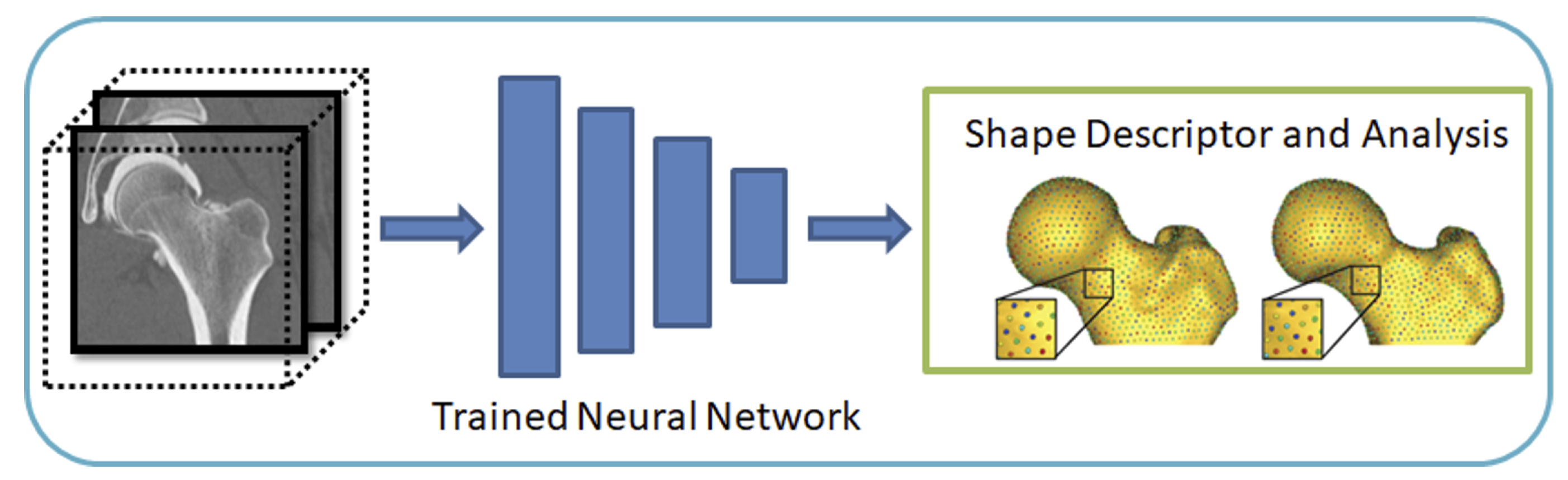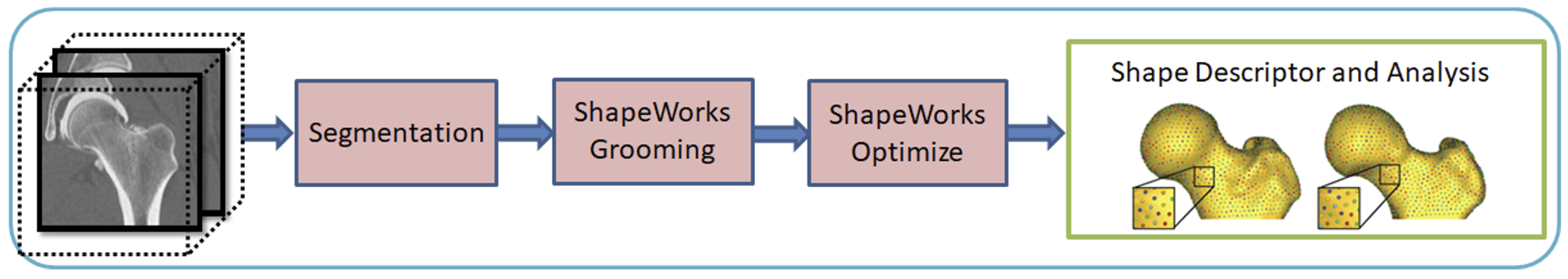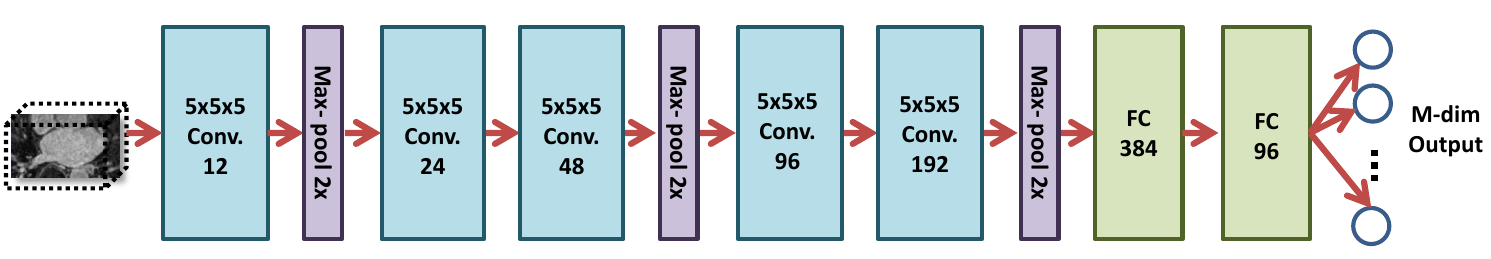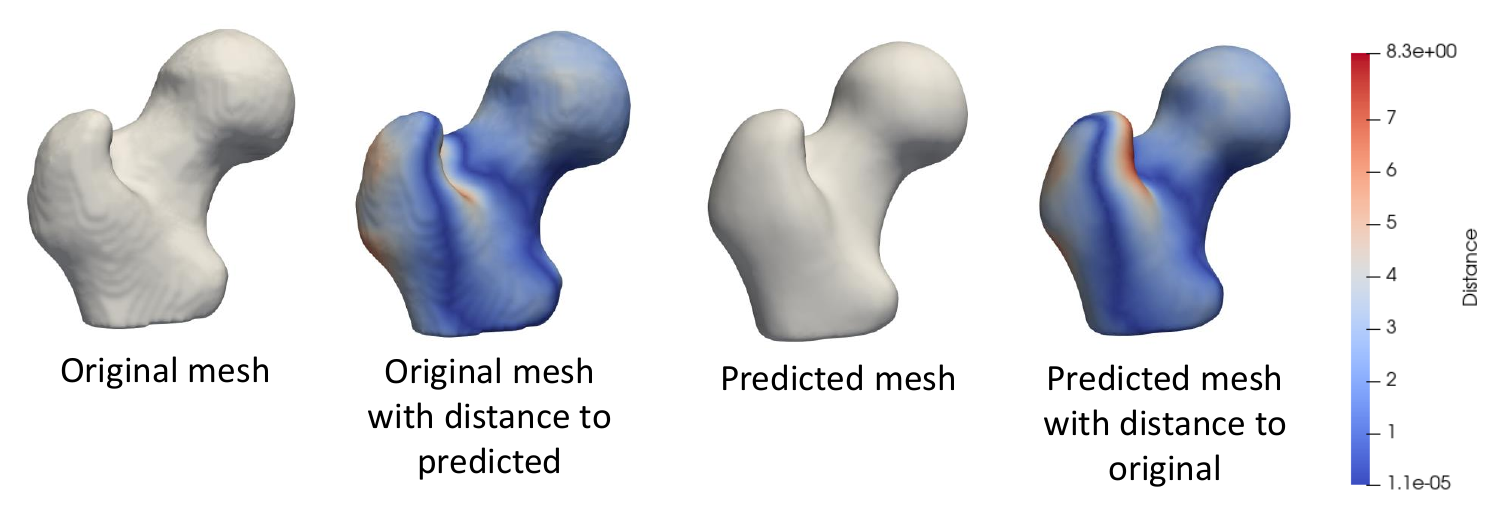# SSMs Directly from Images

DeepSSM is a deep learning framework that estimates statistical representations of shape directly from unsegmented images once trained. DeepSSM includes a data augmentation process and a convolutional neural network (CNN) model. This documentation provides an overview of the DeepSSM process; see relevant papers for a full explanation.

Relevant papers

• Jadie Adams, Riddhish Bhalodia, Shireen Elhabian. Uncertain-DeepSSM: From Images to Probabilistic Shape Models. In MICCAI-ShapeMI, Springer, Cham, 2020.
• Riddhish Bhalodia, Shireen Elhabian, Ladislav Kavan, and Ross Whitaker. DeepSSM: a deep learning framework for statistical shape modeling from raw images. In MICCAI-ShapeMI, pp. 244-257. Springer, Cham, 2018.
• Riddhish Bhalodia, Anupama Goparaju, Tim Sodergren, Alan Morris, Evgueni Kholmovski, Nassir Marrouche, Joshua Cates, Ross Whitaker, Shireen Elhabian. Deep Learning for End-to-End Atrial Fibrillation Recurrence Estimation. Computing in Cardiology (CinC), 2018.

## What is DeepSSM?

The input to the DeepSSM network is unsegmented 3D images of the anatomy of interest, and the output is the point distribution model (PDM).DeepSSM requires training examples of image/PDM pairs that are generated via the traditional Shapeworks grooming and optimization pipeline or other particle distribution models. Once the network has been trained on these examples, it can predict the PDM of unseen examples given only images of the same anatomy/object class, bypassing the need for labor-intensive segmentation, grooming, and optimization parameter tuning.## Why DeepSSM?

The benefits of the DeepSSM pipeline include:

• Less Labor: DeepSSM does not require segmentation, only a bounding box about where the anatomy of interest lies in the image.
• End-to-end: Does not require separate grooming and optimization steps; it is an end-to-end process. This also reduces memory requirement as images do not need to be saved after intermediate grooming steps.
• Faster Results: Once a DeepSSM network has been trained, it can be used to predict the shape model on a new image in seconds on a GPU.

The DeepSSM network is implemented in PyTorch and requires a GPU to run efficiently.

## DeepSSM Steps

### 1. Data Augmentation

The first step to creating a DeepSSM model is generating training data. Deep networks require thousands of training instances and since medical imaging data is typically limited, data augmentation is necessary. The data augmentation process is described here:  Data Augmentation for Deep Learning.

The data augmentation process involves reducing the PDM's to a low-dimensional space via Principal Component Analysis (PCA), preserving a chosen percentage of the variation. The PCA scores are saved and used as the target output for DeepSSM prediction. The PCA scores are deterministically mapped back to the PDM (i.e., shape space) using the eigenvalues and vectors once the DeepSSM model makes a prediction.

### 2. Creation of Data Loaders

The next step is to reformat the data (original and augmented) into PyTorch tensors. 80% of the data is randomly selected to be training data, and the remaining 20% of the data is used as a validation set. The input images are whitened and turned into tensors. They can also be optionally downsampled to a smaller size to allow for faster training. The corresponding PCA scores are also normalized or whitened to avoid DeepSSM learning to favor the primary modes of variation and are then turned to tensors. PyTorch data loaders are then created with a batch size specified by the user.

### 3. Training

PyTorch is used in constructing and training DeepSSM. The network architecture is defined to have five convolution layers followed by two fully connected layers, as illustrated in the figure below. Parametric ReLU activation is used, and the weights are initialized using Xavier initialization. The network is trained for the specified number of epochs using Adam optimization to minimize the L2 loss function with a learning rate of 0.0001. The average training and validation error are printed and logged each epoch to determine convergence.### 4. Testing

The trained model is then used to predict the PCA score from the images in the test set. These PCA scores are then un-whitened and mapped back to the particle coordinates using the eigenvalues and eigenvectors from PCA. Thus a PDM is acquired for each test image.

### 5. Evaluation

To evaluate the accuracy of DeepSSM output, we compare a mesh created from the ground truth segmentation to a mesh created from the predicted PDM. To obtain the original mesh, we use the ShapeWorks MeshFromDistanceTransforms command to the isosurface mesh from the distance transform created from the true segmentation. To obtain the predicted mesh, we use the ShapeWorks ReconstructSurface command with the mean and predicted particles to reconstruct a surface.

We then compare the original mesh to the predicted mesh via surface-to-surface distance. To find the distance from the original to the predicted, we consider each vertex in the original and find the shortest distance to the predicted mesh's surface. This process is not symmetric as it depends on the vertices of one mesh, so the distance from the predicted to the original will be slightly different. We compute the Hausdorff distance that takes the max of these vertex-wise distances to return a single value as a measure of accuracy. We also consider the vertex-wise distances as a scalar field on the mesh vertices and visualize them as a heat map on the surface. This provides us with a way of seeing where the predicted PDM was more or less accurate.## Using the DeepSSM Python Package

The ShapeWorks DeepSSM package, DeepSSMUtils, is installed to the ShapeWorks anaconda environment when conda_installs.sh is run.

Activate shapeworks environment

Each time you use ShapeWorks and/or its Python packages, you must first activate its environment using the conda activate shapeworks command on the terminal.

To use the DeepSSMUtils package, make sure you have the shapeworks conda environment is activated and add the following import to your Python code:

import DeepSSMUtils


### Get train and validation torch loaders

This function turns the original and augmented data into training and validation torch loaders. The data provided is randomly split so that 80% is used in the training set and 20% is used in the validation set.

DeepSSMUtils.getTrainValLoaders(out_dir, data_aug_csv, batch_size=1, down_sample=False)


Input arguments:

• out_dir: Path to the directory to store the torch loaders.
• data_aug_csv: The path to the csv containing original and augmented data, which is the output when running data augmentation as detailed in Data Augmentation for Deep Learning.
• batch_size: The batch size for training data. The default value is 1.
• down_sample: If true, the images will be downsampled to a smaller size to decrease the time needed to train the network. If false, the full image will be used. The default is false.

This function turns the provided data into a test torch loader.

DeepSSMUtils.getTestLoader(out_dir, test_img_list, down_sample)


Input arguments:

• out_dir: Path to the directory to store the torch loader.
• test_img_list: A list of paths to the images that are in the test set.
• down_sample: If true, the images will be downsampled. If false, the full image will be used. This should match what is done for the training and validation loaders. The default is false.

### Train DeepSSM

This function defines a DeepSSM model and trains it on the data provided.

DeepSSMUtils.trainDeepSSM(loader_dir, parameters, out_dir)


Input arguments:

• loader_dir: Path to directory where train and validation torch loaders are.
• parameters: A dictionary of network parameters with the following keys.
• epochs: The number of epochs to train for.
• learning_rate: The value of the learning rate.
• val_freq: How often to evaluate on the validation set. 1 means test on the validation set every epoch, 2 means every other epoch, and so on.
• out_dir: Directory to save the model and training/validation logs.

### Test DeepSSM

This function gets predicted shape models based on the images provided using a trained DeepSSM model.

DeepSSMUtils.testDeepSSM(out_dir, model_path, loader_dir, PCA_scores_path, num_PCA)


Input arguments:

• out_dir: Path to directory where predictions are saved.
• model_path: Path to train DeepSSM model.
• loader_dir: Path to the directory containing test torch loader.
• PCA_scores_path: Path to eigenvalues and eigenvectors from data augmentation that are used to map predicted PCA scores to particles.
• num_PCA: The number of PCA scores the DeepSSM model is trained to predict.

### Analyze Results

This function analyzes the shape models predicted by DeepSSM by comparing them to the true segmentation.

DeepSSMUtils.analyzeResults(out_dir, DT_dir, prediction_dir, mean_prefix)


Input arguments:

• out_dir: Path to the directory where meshes and analysis should be saved.
• DT_dir: Path to the directory containing distance transforms based on the true segmentations of the test images.
• prediction_dir: Path to the directory containing predicted particle files from testing DeepSSM.
• mean_prefix: Path to the mean particle and mesh files for the dataset.